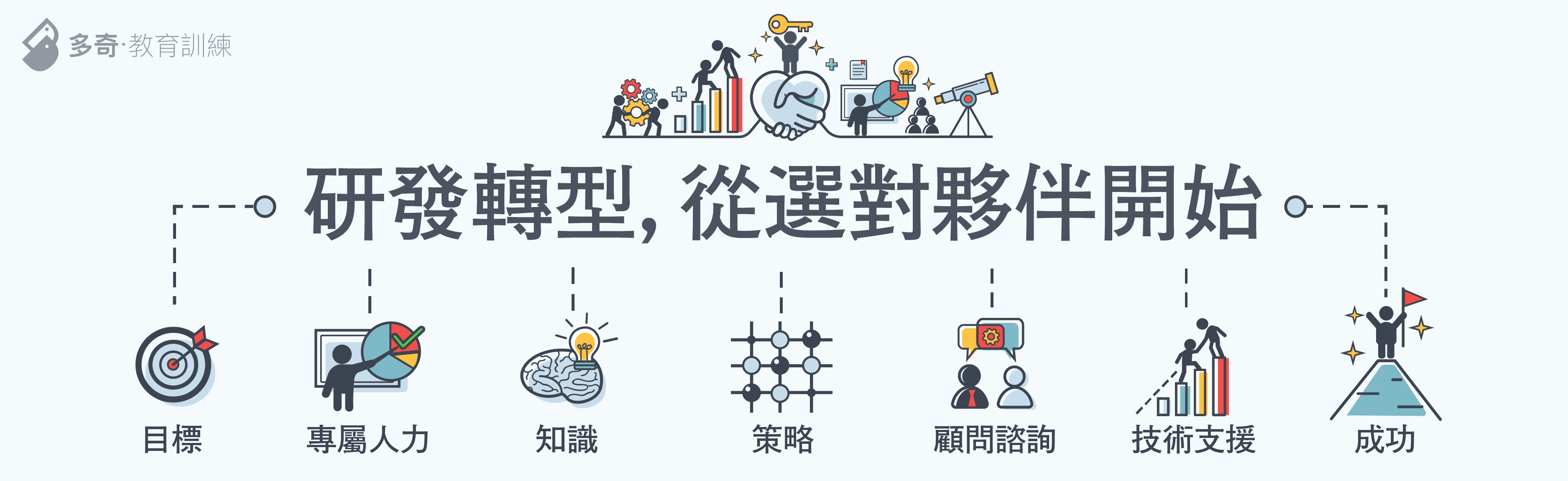C# 中的進制轉換 | The Will Will Web# The Will Will Web

### 記載著 Will 在網路世界的學習心得與技術分享[code:c#]

int iNum = 253;
string strResult;

strResult = Convert.ToString(iNum, 2);
// 結果：11111101

strResult = Convert.ToString(iNum, 8);
// 結果：375

strResult = Convert.ToString(iNum, 10);
// 結果：253

strResult = Convert.ToString(iNum, 16);
// 結果：fd

[/code]

[code:c#]

int intResult;

intResult = Convert.ToInt32("11111101", 2);
// 結果：253

intResult = Convert.ToInt32("375", 8);
// 結果：253

intResult = Convert.ToInt32("253", 10);
// 結果：253

intResult = Convert.ToInt32("fd", 16);
// 結果：253

[/code]